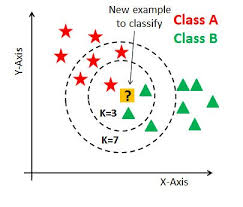2 views

I need to classify some data with (I hope) nearest-neighbor algorithm. I've googled this problem and found a lot of libraries (including PyML, mlPy, and Orange), but I'm unsure of where to start here.

How should I go about implementing k-NN using Python?

by (33.1k points)

You can use Scikit learn for k nearest neighbours (KNN)

KNN algorithm is used for both regression (returns a score) and classification (returns a class label).

Using the scikits.learn k-nearest neighbor module:

>>> import numpy as NP

>>> from sklearn import neighbors as kNN

>>> from sklearn import datasets

>>> data = iris.data

>>> class_labels = iris.target

>>> kNN1 = kNN.NeighborsClassifier()

>>> kNN1.fit(data, class_labels)

NeighborsClassifier(n_neighbors=5, leaf_size=20, algorithm='auto')

K-nearest neighbors require an appropriate similarity metric (Euclidean distance). Scikits.learn includes modules comprised of various distance metrics as well as testing algorithms for the selection of the appropriate one.